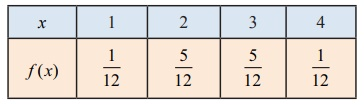Home | | Maths 12th Std | Cumulative Distribution Function from Probability Mass function

# Cumulative Distribution Function from Probability Mass function

Both the probability mass function and the cumulative distribution function of a discrete random variable X contain all the probabilistic information of X.

## Cumulative Distribution Function from Probability Mass function

Both the probability mass function and the cumulative distribution function of a discrete random variable X contain all the probabilistic information of X. The probability distribution of X is determined by either of them. In fact, the distribution function F of a discrete random variable X can be expressed in terms of the probability mass function f(x) of X and vice versa.

### Example 11.7

If the probability mass function f ( x) of a random variable X isfind (i) its cumulative distribution function, hence find (ii) PX  3) and, (iii) PX  2)

### Solution

(i) By definition the cumulative distribution function for discrete random variable is

( x) = P( X≤ x) = ∑xi≤xP( X = xi )Therefore the cumulative distribution function is### Example 11.8

A six sided die is marked ‘1’ on one face, ‘2’ on two of its faces, and ‘3’ on remaining three faces. The die is rolled twice. If X denotes the total score in two throws.(i) Find the probability mass function.

(ii) Find the cumulative distribution function.

(iii) Find P (3≤ X < 6) (iv) Find P ( X  4) .

Solution:

Since X denotes the total score in two throws, it takes on the values 2, 3, 4, 5, and 6.

From the Sample space S, we haveTags : Probability Distributions | Mathematics , 12th Maths : UNIT 11 : Probability Distributions
Study Material, Lecturing Notes, Assignment, Reference, Wiki description explanation, brief detail
12th Maths : UNIT 11 : Probability Distributions : Cumulative Distribution Function from Probability Mass function | Probability Distributions | Mathematics